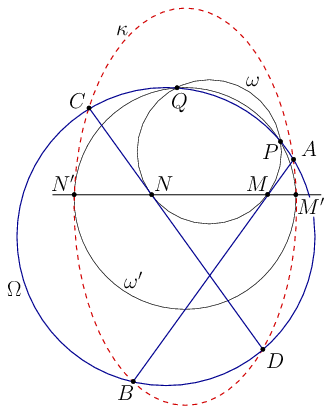Mathematical and Physical Journal
for High Schools
Issued by the MATFUND Foundation
 Already signed up? New to KöMaL?

#Problem A. 685. (December 2016)

A. 685. Let $\displaystyle AB$ and $\displaystyle CD$ be two chords in a circle $\displaystyle \Omega$. Choose a circle $\displaystyle \omega$ tangent to the segments $\displaystyle AB$ and $\displaystyle CD$ at their interior points $\displaystyle M$ and $\displaystyle N$ respectively, and let $\displaystyle \omega$ intersect $\displaystyle \Omega$ at $\displaystyle P$ and $\displaystyle Q$. Suppose that a third circle $\displaystyle \omega'$, distinct from $\displaystyle \Omega$ and $\displaystyle \omega$, and also passing through $\displaystyle P$ and $\displaystyle Q$, meets the line $\displaystyle MN$ at $\displaystyle M'$ and $\displaystyle N'$. Prove that the points $\displaystyle A$, $\displaystyle B$, $\displaystyle C$, $\displaystyle D$, $\displaystyle M'$, and $\displaystyle N'$ lie on a conic section that is tangent to $\displaystyle \omega'$ at $\displaystyle M'$ and $\displaystyle N'$.

(Proposed by: Ilya Bogdanov and Pavel Kozhevnikov, Moscow)

(5 pont)

Deadline expired on January 10, 2017.Solution. Let $\displaystyle f(x)=0$, $\displaystyle g(x)=0$, and $\displaystyle m(x)=0$ be equations (with degrees $\displaystyle 2$, $\displaystyle 2$, and $\displaystyle 1$, respectively) of the circles $\displaystyle \Omega$, $\displaystyle \omega$, and the line $\displaystyle MN$, respectively.

The pencil of conics generated by $\displaystyle g(x)=0$ and $\displaystyle m(x)^2=0$ consists of all conics which have double intersections with $\displaystyle \omega$ at $\displaystyle M$ and $\displaystyle N$; thus the union of the lines $\displaystyle AB$ and $\displaystyle CD$ lies in this pencil, so its equation is

 $\displaystyle g(x)+\alpha m(x)^2=0 \qquad \text{with some nonzero $$\displaystyle \alpha\in\mathbb{R}$}.$$ $\displaystyle {(AB\cup CD)}$

The pencil of circles generated by $\displaystyle \Omega$ and $\displaystyle \omega$ consists of all circles passing through $\displaystyle P$ and $\displaystyle Q$ (including the line $\displaystyle PQ$ as a degenerate case). Thus the circle $\displaystyle \omega'$ lies in this pencil, so its equation is

 $\displaystyle g(x)+\beta f(x)=0 \qquad \text{with some nonzero $$\displaystyle \beta\in\mathbb R$}.$$ $\displaystyle {(\omega')}$

Now consider the conic $\displaystyle \kappa$ with the equation

 $\displaystyle g(x)+\alpha m(x)^2+\beta f(x)=0.$ $\displaystyle {(\kappa)}$

This conic lies in the pencil of conics generated by $\displaystyle AB\cup CD$ and $\displaystyle \Omega$, so $\displaystyle \kappa$ passes though their common points $\displaystyle A$, $\displaystyle B$, $\displaystyle C$, and $\displaystyle D$. On the other hand, this equation is a linear combination of $\displaystyle m(x)^2=0$ and the equation of $\displaystyle \omega'$; thus $\displaystyle \kappa$ has double intersections with $\displaystyle \omega'$ at $\displaystyle M'$ and $\displaystyle N'$, which means that $\displaystyle \kappa$ is tangent to $\displaystyle \omega'$ at $\displaystyle M'$ and $\displaystyle N'$. Hence $\displaystyle \kappa$ is the required conic.

For completeness, notice that the equation $\displaystyle \kappa$ cannot be trivial because the points $\displaystyle P$ and $\displaystyle Q$ do not satisfy it.

Remark. As a corollary, one may obtain the following (known) fact: There exists a circle through $\displaystyle P$ and $\displaystyle Q$ tangent to $\displaystyle AD$ and $\displaystyle BC$, and the points of tangency lie on the line $\displaystyle MN$.

### Statistics:

 3 students sent a solution. 5 points: Bukva Balázs, Matolcsi Dávid, Williams Kada.

Problems in Mathematics of KöMaL, December 2016